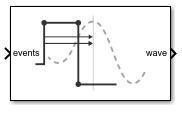# Slew Rate

Model amplitude, rise and fall times, and propagation delay of logic gates

• Library:
• Mixed-Signal Blockset / Utilities

•## Description

The Slew Rate block converts a logical signal to a signal with user-defined finite slew rate and propagation delay.

## Ports

### Input

expand all

Variable step, event driven input signal, specified as a scalar. The signal at events port comes from the output of a logic gate such as Variable Pulse Delay block.

Data Types: `single` | `double` | `int8` | `int16` | `int32` | `int64` | `uint8` | `uint16` | `uint32` | `uint64` | `Boolean`

### Output

expand all

Fixed step, uniformly sampled output signal, returned as a scalar. You can define the finite slew rate and propagation delay for the signal at the wave port.

Data Types: `double`

## Parameters

expand all

Defines how to calculate output step size.

• Choose `Default` to calculate Output step size calculation based on rise/fall time. The Output sample interval (ΔT) is given by .

• Choose `Advanced` to calculate Output step size calculation based on frequency of interest. The Output sample interval (ΔT) is given by .

#### Programmatic Use

• Use `get_param(gcb,'DefaultOrAdvanced')` to determine how the output step size is being calculated.

Maximum frequency of interest at output, specified as a scalar in Hz. Maximum frequency of interest is used to calculate Output sample interval and Minimum 20%-80% rise/fall time.

#### Dependencies

This parameter is only available when `Advanced` is selected for Output step size calculation.

#### Programmatic Use

• Use `get_param(gcb,'MaxFreqInterest')` to view the current value of Maximum frequency of interest.

• Use `set_param(gcb,'MaxFreqInterest',value)` to set Maximum frequency of interest to a specific value.

Output sample interval, specified as a scalar in s. This parameter is nontunable.

The Output sample interval (ΔT) is given by .

#### Dependencies

This parameter is only available when `Advanced` is selected for Output step size calculation.

#### Programmatic Use

• Use `get_param(gcb,'OutputSampleTime')` to view the current value of Output sample interval.

• Use `set_param(gcb,'OutputSampleTime',value)` to set Output sample interval to a specific value.

Minimum rise/fall time required at the output for meaningful simulation, specified as a scalar in ps. This is a nontunable parameter.

#### Dependencies

This parameter is only available when `Advanced` is selected for Output step size calculation.

#### Programmatic Use

• Use `get_param(gcb,'ConversionRiseFall')` to view the current value of Minimum 20%-80% rise/fall time.

• Use `set_param(gcb,'ConversionRiseFall',value)` to set Minimum 20%-80% rise/fall time to a specific value.

Time required for signal to change from 20% to 80% in a full amplitude edge at the output, specified as a scalar.

#### Programmatic Use

• Use `get_param(gcb,'RiseTime')` to view the current value of Rise/fall time.

• Use `set_param(gcb,'RiseTime',value)` to set Rise/fall time to a specific value.

Slew rate for the 20%-80% edge at the output, specified as a scalar. This parameter is nontunable.

#### Programmatic Use

• Use `get_param(gcb,'RisingSlewRate')` to view the current value of Slew rate for 20%-80% edge of unit amplitude signal.

• Use `set_param(gcb,'RisingSlewRate',value)` to set Slew rate for 20%-80% edge of unit amplitude signal to a specific value.

Minimum propagation delay for meaningful simulation, specified as a scalar. This parameter is nontunable.

Minimum propagation delay is calculated from Maximum frequency of interest where .

#### Programmatic Use

• Use `get_param(gcb,'ConversionDelay')` to view the current value of Minimum propagation delay.

• Use `set_param(gcb,'ConversionDelay',value)` to set Minimum propagation delay to a specific value.

Propagation delay of a rising edge, specified as a scalar in s. Propagation delay is measured at a threshold equal to the half of the amplitude.

#### Programmatic Use

• Use `get_param(gcb,'RisepropDelay')` to view the current value of Propagation delay.

• Use `set_param(gcb,'RisePropDelay',value)` to set Propagation delay to a specific value.

Select to enable increased buffer size during the simulation. This increases the buffer size of all the blocks in the PLL model that belong to the Mixed-Signal Blockset™/Utilities Simulink® library. By default, this option is deselected.

Buffer size of all the blocks in the PLL model that belong to the Mixed-Signal Blockset/Utilities Simulink library.

Selecting different simulation solver or sampling strategies can change the number of input samples needed to produce an accurate output sample. Set the Buffer size to a large enough value so that the input buffer contains all the input samples required.

#### Dependencies

This parameter is only available when the Enable increased buffer size option is selected.

#### Programmatic Use

• Use `get_param(gcb,'NBuffer')` to view the current value of Buffer size.

• Use `set_param(gcb,'NBuffer',value)` to set Buffer size to a specific value.